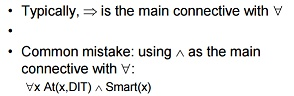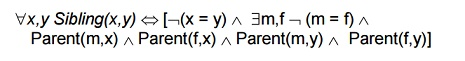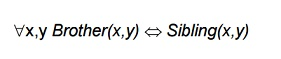Home | | Artificial Intelligence | Predicate Calculus

# Predicate Calculus

Whereas propositional logic assumes the world contains facts, first-order logic (like natural language) assumes the world contains

Predicate Calculus

First-order logic

Whereas propositional logic assumes the world contains facts,

first-order logic (like natural language) assumes the world contains

Objects: people, houses, numbers, colors, baseball games, wars, …

Relations: red, round, prime, brother of, bigger than, part of, comes between, …

Syntax of FOL: Basic elements

•        Constants   TaoiseachJohn, 2, DIT,...

•        Predicates   Brother, >,...

•        Functions   Sqrt, LeftLegOf,...

•        Variables    x, y, a, b,...

•        Connectives         ,•        Equality     =

•        Quantifiers ,Atomic sentences

Atomic sentence =                            predicate (term1,...,termn)

or term1 = term2

Term                              =                 function (term1,...,termn)

or constant or variable

E.g., Brother(TaoiseachJohn,RichardTheLionheart) > (Length(LeftLegOf(Richard)), Length(LeftLegOf(TaoiseachJohn)))

Complex sentences

Complex sentences are made from atomic sentences using connectivesTruth in first-order logic

Sentences are true with respect to a model and an interpretation

Model contains objects (domain elements) and relations among them

Interpretation specifies referents for

constant symbols →      objects

predicate symbols         →      relations

function symbols →      functional relations

An atomic sentence predicate(term1,...,termn) is true iff the objects referred to by term1,...,termn

are in the relation referred to by predicate

Universal quantificationRoughly speaTaoiseach, equivalent to the conjunction of instantiations of P

At(TaoiseachJohn,DIT) -- >  Smart(TaoiseachJohn)

At(Richard,DIT) -- > Smart(Richard)

At(DIT,DIT)         -- > Smart(DIT)

A common mistake to avoidmeans “Everyone is at DIT and everyone is smart”

Existential quantificationRoughly speaTaoiseach, equivalent to the disjunction of instantiations of P

At(TaoiseachJohn,DIT) -- > Smart(TaoiseachJohn)

At(Richard,DIT)   -- > Smart(Richard)

At(DIT,DIT)         -- > Smart(DIT)

Another common mistake to avoidProperties of quantifiersEquality

term1 = term2 is true under a given interpretation if and only if term1 and term2 refer to the same object

E.g., definition of Sibling in terms of Parent:Using FOL

The kinship domain:

Brothers are siblingsOne's mother is one's female parent“Sibling” is symmetric

Knowledge engineering in FOL

·        Assemble the relevant knowledge

·        Decide on a vocabulary of predicates, functions, and constants

·        Encode general knowledge about the domain

·        Encode a description of the specific problem instance

·        Pose queries to the inference procedure and get answers

·        Debug the knowledge base

Summary

First-order logic:

objects and relations are semantic primitives

syntax: constants, functions, predicates, equality, quantifiers

Semantics for Predicate Calculus

An interpretation over D is an assignment of the entities of D to each of the constant, variable, predicate and function symbols of a predicate calculus expression such that:

1: Each constant is assigned an element of D

2: Each variable is assigned a non-empty subset of D;(these are the allowable substitutions for that variable)

3: Each predicate of arity n is defined on n arguments from D and defines a mapping from Dn into {T,F}

4: Each function of arity n is defined on n arguments from D and defines a mapping from Dn into D

The meaning of an expression

Given an interpretation, the meaning of an expression is a truth value assignment over the interpretation.

Truth Value of Predicate Calculus expressions

Assume an expression E and an interpretation I for E over a non empty domain D. The truth value for E is determined by:

The value of a constant is the element of D assigned to by I

The value of a variable is the set of elements assigned to it by I

More truth values

The value of a function expression is that element of D obtained by evaluating the function for the argument values assigned by the interpretation

The value of the truth symbol “true” is T

The value of the symbol “false” is F

The value of an atomic sentence is either T or F as determined by the interpretation I

Similarity with Propositional logic truth values

The value of the negation of a sentence is F if the value of the sentence is T and F otherwise

The values for conjunction, disjunction ,implication and equivalence are analogous to their propositional logic counterparts

Universal Quantifier

The value forIs T if S is T for all assignments to X under I, and F otherwise

Existential Quantifier

The value forIs T if S is T for any assignment to X under I, and F otherwise

Some Definitions

A predicate calculus expressions S1 is satisfied.

Definition If there exists an Interpretation I and a variable assignment under I which returns a value T for S1 then S1 is said to be satisfied under I.

S is Satisfiable if there exists an interpretation and variable assignment that satisfies it: Otherwise it is unsatisfiable

A set of predicate calculus expressions S is satisfied.

Definition For any interpretation I and variable assignment where a value T is returned for every element in S the the set S is said to be satisfied,

A set of expressions is satisfiable if and only if there exist an intrepretation and variable assignment that satisfy every element

If a set of expressions is not satisfiable, it is said to be inconsistent

If S has a value T for all possible interpretations , it is said to be valid

A predicate calculus expressions S1 is satisfied.

Definition If there exists an Interpretation I and a variable

assignment under I which returns a value T for S1 then S1 is

said to be satisfied under I.

A set of predicate calculus expressions S is satisfied.

Definition For any interpretation I and variable assignment

where a value T is returned for every element      in S the the set

S is said to be satisfied,

An inference rule is complete.

Definition If all predicate calculus expressions X that logically

follow from a set of expressions, S can be produced using the

inference rule , then the inference rule is said to be complete.

A predicate calculus expression X logically follows from a set S of predicate calculus expressions .

For any interpretation I and variable assignment where S is satisfied, if X is also satisfied under the same interpretation and variable assignment then X logically follows from S.

Logically follows is sometimes called entailment

Soundness

An inference rule is sound.

If all predicate calculus expressions X produced using the inference rule from a set of expressions, S logically follow from S then the inference rule is said to be sound.

Completeness

An inference Rule is complete if given a set S of predicate calculus expressions, it can infer every expression that logically follows from S

Study Material, Lecturing Notes, Assignment, Reference, Wiki description explanation, brief detail
Artificial Intelligence(AI) : Representation of Knowledge : Predicate Calculus |